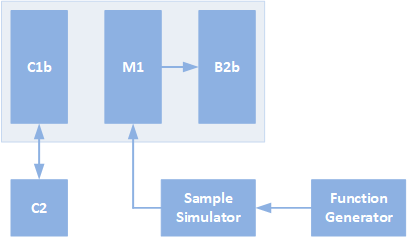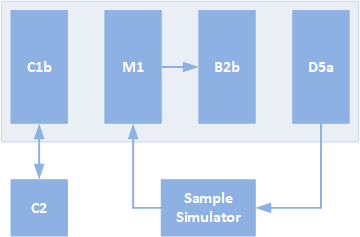# B2b

Example for the B2b, 2 channel 24-bit ADC module. The module contains the same ADCs as the D4 and is identical in hardware to the D4b module: the only difference being the absence of connectors on the front of the module. Both of them differ to the D4 by the addition of an ARM microcontroller. This allows for operations where exact timing and local storage is needed.

#### SPI Rack setup

To use the D5b module, we need to import both the D5b_module and the SPI_rack module from the spirack library. All the communication with the SPI Rack runs through the SPI_rack object which communicates through a virtual COM port. This COM port can only be open on one instance on the PC. Make sure you close the connection here before you can use it somewhere else.

We also import the logging library to be able to display the logging messages; numpy for data manipulation; scipy for the FFT analysis and plotly for visualistation.

from spirack import SPI_rack, B2b_module, D5a_module, D4b_module

import logging

from time import sleep
from tqdm import tqdm_notebook

import numpy as np
from scipy import signal

from plotly.offline import init_notebook_mode, iplot, plot
import plotly.graph_objs as go

init_notebook_mode(connected=True)

logger = logging.getLogger(__name__)
logging.basicConfig(level=logging.INFO)


Open the SPI rack connection and unlock the controller. This is necessary after bootup of the controller module. If not unlocked, no communication with the modules can take place. The virtual COM port baud rate is irrelevant as it doesn’t change the actual speed. Timeout can be changed, but 1 second is a good value.

COM_port = 'COM4' # COM port of the SPI rack
COM_speed = 1e6   # Baud rate, not of much importance
timeout = 1       # Timeout value in seconds

spi_rack = SPI_rack(COM_port, COM_speed, timeout)
spi_rack.unlock() # Unlock the controller to be able to send data to the rack


Read back the version of the microcontroller software. This should return 1.6 or higher to be able to use the B2b properly. Als read the temperature and the battery voltages through the C1b, this way we verify that the connection with the SPI Rack is working.

print('Version: ' + spi_rack.get_firmware_version())
print('Temperature: {:.2f} C'.format(spi_rack.get_temperature()))
battery_v = spi_rack.get_battery()
print('Battery: {:.3f}V, {:.3f}V'.format(battery_v, battery_v))


Create a new B2b module object at the correct module address using the SPI object. If we set calibrate=True, the module will run a calibration routine at initialisation. This takes about 2 seconds, during which the python code will stall all operations.

To see that we have a connection, we read back the firmware version.

B2b = B2b_module(spi_rack, module=4, calibrate=False)
print("Firmware version: {}".format(B2b.get_firmware_version()))


### FFT

One useful application of the B2b module is to find interference. The module can be set to run at a high sample rate and store a trace in the local memory. If we run an FFT on this data, we will be able to see all kinds of interference signals present in our setup. To demonstrate this, we will use the measurement setup as shown in the image below.We will set the function generator to generate a 500 Hz signal of ±100mV and run it through a sample simulator at 10MΩ. The B2b will be connected to a current measurement module, in this case an old M1 I-measure module that was lying around.

First we have to configure the B2b for acquiring long traces of data.

#### Configuring the B2b for FFT

The B2b module can run from either a local (inside the module) clock or a user provided clock from the backplane. This backplane clock should be 10 MHz and either a square or a sine wave. If there are more modules with microcontrollers in the rack, and they need to run synchronously, it is recommended to use the backplane clock. For a single module it is fine to run it using the local clock.

If the external clock is selected but not present, the user will get an ERROR to the logger and the microcontroller will keep running on the internal clock. Never turn off the external clock if the microcontroller is running on it. This will stop the module from functioning.

In this example we will use the internal clock:

B2b.set_clock_source('internal')
print("Clock source: {}".format(B2b.get_clock_source()))


To get the B2b module to do anything, it needs to be triggered. There are three ways of triggering the module:

• Software trigger
• Controller generated trigger
• D5b generated trigger

The software trigger is generated by the PC, which means that the timing is not very exact. Depending on the user application, this might be acceptable. As an example, it would be perfectly fine for finding interference: take a long trace and run an FFT on the data.

The controller generated trigger eliminates the issue of the software trigger: the timing is now handled by the microcontroller in the controller module. This allows for exact alignment with other operations. There are two ways the controller can generate a trigger: directly by a PC command, or synchronous with another SPI command. This last one is the most interesting, you can for example generate a trigger at the moment you’re sending a message to update the voltage on the D5a module. This allows for synchronous measurements and takes the PC out of the picture. The controller generated triggers will be on the backplane for all modules to see, so it allows the user to trigger multiple modules at once.

Finally there is also the D5b generated trigger: it generates a trigger everytime it toggles the output (in toggling mode). This allows for lock-in type of measurements. For more information on that, see the lock-in example notebook.

In this notebook we will be using both the software trigger and the controller generated trigger. First we’ll use the software trigger. To do this, we’ll set the trigger input to ‘None’ to make the B2b ignore the trigger lines on the backplane. We only expect one trigger, and we don’t need any hold off time. This is a dead time which the B2b will wait after the trigger before it starts measuring. It can be set with a resolution of 100 ns.

B2b.set_trigger_input("None")
B2b.set_trigger_amount(1)
B2b.set_trigger_holdoff_time(0)


We’ll measure on channel one (zero in software), so we need to enable it. For the FFT we’ll take 10000 measurements with filter setting 0 on the sinc5 filter. This will give a datarate of 50 kSPS and a resolution of 16.8 bit. For details on all the filter settings, see the excel sheet for the D4_filter.

filter_type = 'sinc5'
filter_setting = 0

B2b.set_sample_amount(0, 10000)
B2b.set_filter_type(0, filter_type)
B2b.set_filter_rate(0, filter_setting)


#### Measurement and plotting

To start a measurement we trigger the B2b via software and keep checking if the module is done measuring.

B2b.software_trigger()

while B2b.is_running():
sleep(0.1)



We use the periodogram from scipy, which will give the power spectral density. Before we do that we have to take the gain of the M1f module into account. It has a gain of 10 MV/A and a postgain of 10.

#Calculate periodogram
T = B2b.sample_time[filter_type][filter_setting]
fs = 1/T

gain = 10*10e6

#Plot the FFT data
pldata0 = go.Scattergl(x=f0, y=np.sqrt(Pxx_den0), mode='lines+markers', name='ADC1')
plot_data = [pldata0]

layout = go.Layout(
title = dict(text='Spectral Density'),
xaxis = dict(title=r'$\text{Frequency [Hz]}$', type='log'),
yaxis = dict(title=r'$\text{PSD [} \text{A/}\sqrt{\text{Hz}} \text{]}$')
)

fig = go.Figure(data=plot_data, layout=layout)
iplot(fig)


### D5a sweep

We’re now gonna perform a sweep with the D5a and measure synchronously with the B2b module. As the timing of the D5a updates is handled by the PC, the time between the sweep steps is not going to be very accurate. This same issue would arise if we used the software trigger of the B2b. To work around this, we will trigger the B2b using the controller trigger.

The measurement setup is displayed in the figure below.Create a new D5a module object at the correct module address using the SPI object. By default the module resets the output voltages to 0 Volt. Before it does this, it will read back the current value. If this value is non-zero it will slowly ramp it to zero. If reset_voltages = False then the output will not be changed.

D5a = D5a_module(spi_rack, module=2, reset_voltages=True)


To get nice equidistant voltage steps, we will use integer multiples of the smallest step the DAC can do in the current range setting.

smallest_step = D5a.get_stepsize(0)
sweep_voltages = np.arange(-3000*smallest_step, 3001*smallest_step, 100*smallest_step)

print('Smallest step: {0:.3f} uV'.format(smallest_step*1e6))
print('Start voltage: {0:.4f} V. Stop voltage: {0:.4f} V'.format(sweep_voltages, sweep_voltages[-1]))
print('Sweep length: {} steps'.format(len(sweep_voltages)))


We now have to tell the B2b module to look out for the controller trigger, with an amount equal to the sweep length. Additionally we will also set a holdoff time of 1ms. This to compensate for any delays through the circuit (due to line length and/or filters).

B2b.set_trigger_input("Controller")
B2b.set_trigger_amount(len(sweep_voltages))
B2b.set_trigger_holdoff_time(10e-3)


We will keep the filter at sinc5, but the rate at 10: a data rate of 1ksps with a settling time of 1 ms. This gives us a resolution of 20.7 bits. The sample_amount is now set to one: only one sample is taken per trigger.

filter_type = 'sinc5'
filter_setting = 10

B2b.set_sample_amount(0, 1)
B2b.set_filter_type(0, filter_type)
B2b.set_filter_rate(0, filter_setting)


Here we see how we can synchronise the updating of the DAC with the triggering of the B2b module. Before we set the net output voltage, we arm the spi_rack controller. This means that it will send a trigger on the next SPI command it receives: in this case the D5a set_voltage command. We’ll then wait a little bit to make sure measurement and settling is done, and go on to the next step.

for value in tqdm_notebook(sweep_voltages):
spi_rack.trigger_arm()
D5a.set_voltage(0, value)
while B2b.is_running():
sleep(1e-3)



Compensating for the gain of the M1 (a factor 10e6), we get the IV curve for our ‘sample’. In this case the sample simulator was set to a series resistance of 10 MOhm with all capacitors at minimum value.

gain = 10e6

plot_data = [pldata]

layout = go.Layout(
title = dict(text='10 MOhm IV Curve'),
xaxis = dict(title='D5a voltage (V)'),
yaxis = dict(title='Current (A)')
)

fig = go.Figure(data=plot_data, layout=layout)
iplot(fig)


When done with this example, it is recommended to close the SPI Rack connection. This will allow other measurement scripts to access the device.

spi_rack.close()